In an engineering design, Bayesian estimation uses subjective judgement. The estimation is an estimated value that decreases the posterior expected value of a loss function. Also, it increases the posterior expected value of utility function.

For Bayesian estimation, for discrete cases, if the parameter takes a value of, where i=1, 2,….,n, with probability  and let be the resultant outcome.

Therefore, by Bayes’ theorem we get,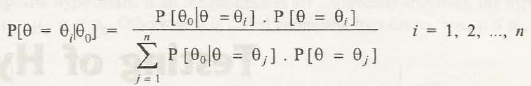P where i=1, 2, ….., n.

Thus, as a result, the expected value of 8 is known as Bayesian estimator of the parameter, that is,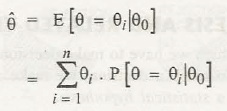With this, the following can be obtained,

P

If the case be continuous, then let 8 be the random variable of the parameter of distribution denoted by the density function f’(.

Therefore, P [< θ <  + ∆θ] = f'() . ∆θ, where i=1, 2, …, n.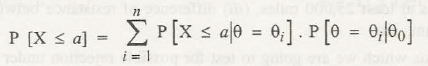If θ0 is the observed experimental outcome, then

f” () ∆θwhere i=1, 2, 3,…., n.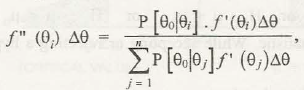In the limit, f”(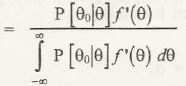Therefore, the Bayesian estimator is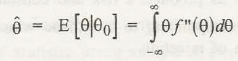This can be used to calculate P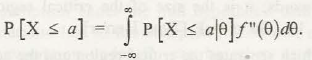### Customer Reviews

My Homework Help
Rated 5.0 out of 5 based on 510 customer reviews at